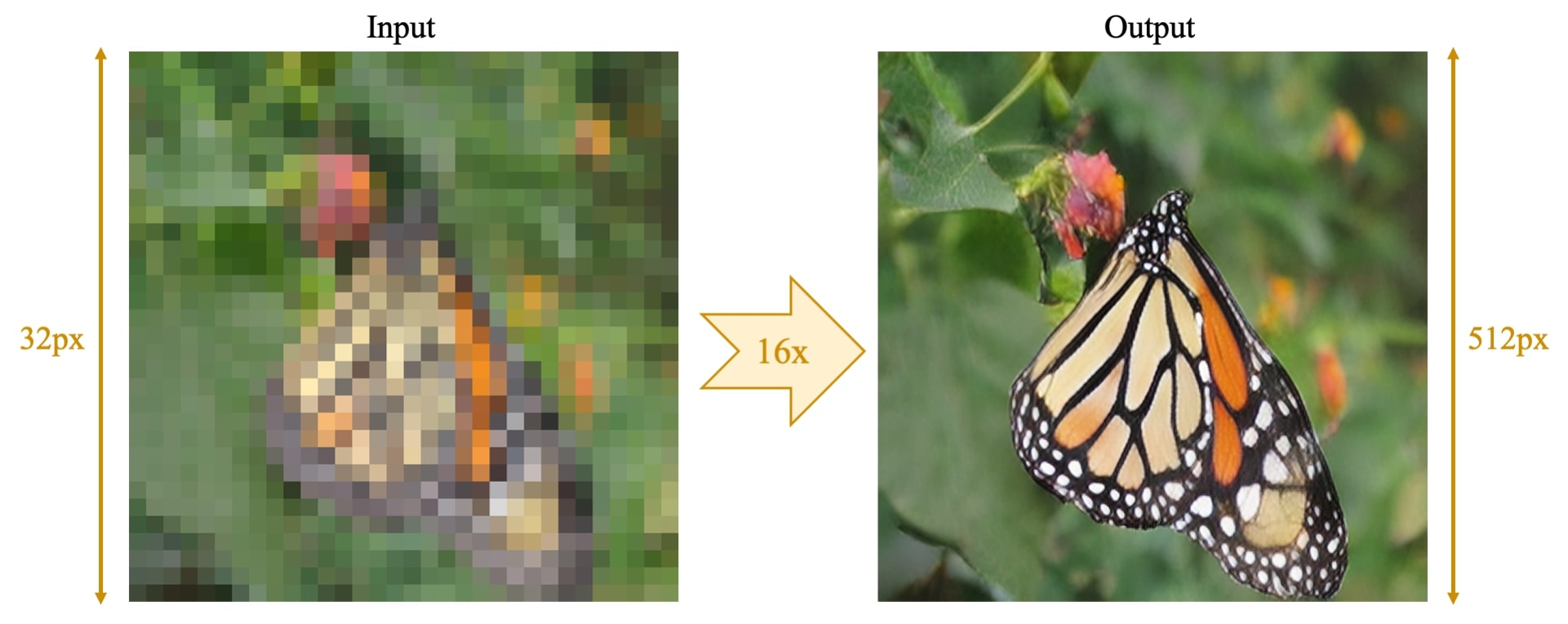# Generating Unobserved Alternatives

A Case Study through Super-Resolution and Decompression

Shichong Peng

Simon Fraser University & University of California, San Diego

Ke Li

Simon Fraser University, Google & Institute for Advanced Study

### Links## Model

The model is the same as a generator in a conditional GAN. However, unlike a conditional GAN, the model avoids mode collapse and can generate multiple outputs for the same input.

## Method

The model is trained with Implicit Maximum Likelihood Estimation (IMLE). The objective function is non-adversarial and aims to cover all modes. It takes the following form (please hover over the coloured letters for descriptions):

$$\min_{\texttip{\color{blue}{\theta}}{Generator Parameters}}\mathbb{E}_{\texttip{\color{red}{\mathbf{z}_{1},\ldots,\mathbf{z}_{m}}}{Latent Code Samples} \sim \texttip{\color{orange}{\mathcal{N}(0, \mathbf{I})}}{Standard Normal Distribution}}\left[\sum_{i=1}^{\texttip{\color{purple}{n}}{Number Of Real Data Points}}\min_{j\in \{1,\ldots,\texttip{\color{purple}{m}}{Number Of Generated Samples}\}}\texttip{\color{brown}{d}}{Distance Metric}(\texttip{\color{blue}{F_{\theta}}}{Generator}(\texttip{\color{red}{\mathbf{z}_{j}}}{Latent Code Sample}), \texttip{\color{green}{\mathbf{y}_{i}}}{Real Data})\right]$$

The animation below shows how it works:

## 16x Super-Resolution

We use our method to increase the width and height of images by a factor of 16x. Toggle for our results (HyperRIM) and those of 2xESRGAN, which is a leading GAN-based 4x super-resolution method applied twice.

## Image Decompression

We use our method to recover a plausible image from a badly compressed image. Toggle for our results (HyperRIM) and those of Pix2Pix.

## IMLE vs GAN

IMLE trains a generator without a discriminator. It has two key differences with GANs: it avoids mode collapse and training instability. The animation below shows what happens when training a GAN.

As shown above, a GAN encourages every generated sample to be similar to a real data point. On the other hand, IMLE flips the direction: it instead encourages every real data point to have a similar generated sample.

#### Mode Collapse

Below is a comparison of the behaviours of GAN and IMLE over the course of training. Real data points are shown as blue crosses and the probability density of generated samples is shown as a heatmap.

GAN

IMLE

As shown above, the GAN usually generates data points at the bottom and largely ignores the data points at the top. In comparison, IMLE can generate all data points with similar frequency.

#### Stable Training

Because IMLE uses a non-adversarial objective, it trains stably.

The output is shown on the left and the loss over time is shown on the right. The output quality improves steadily over the course of training.

#### Recall Evaluation by Inverting Generator

The ideal generator should be able to reconstruct the observed real image from some latent code. We keep the generator fixed and use gradient descent to find a latent code that reproduces the real image.

2xESRGAN

HyperRIMObserved Real Image

As shown above, our method (HyperRIM) successfully reconstructs the real image, whereas 2xESRGAN fails to do so.